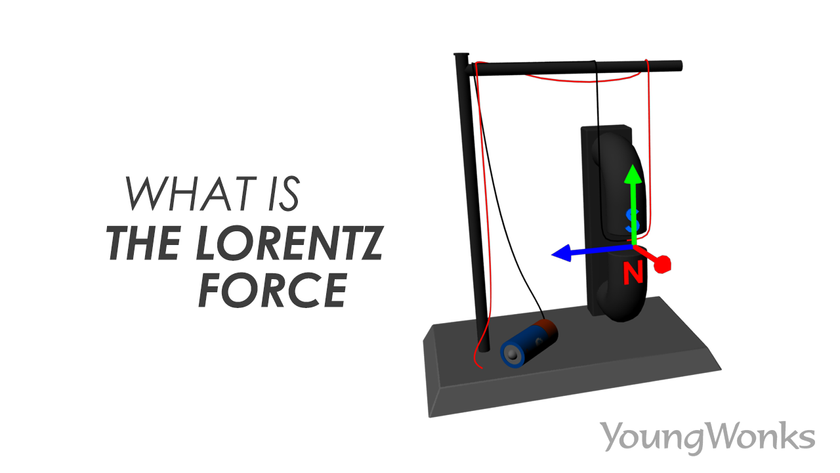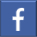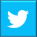# What is Lorentz Force?

## A look at Lorentz Force and its applicationsAug 21, 2018    By Team YoungWonks *

What is Lorentz Force?

Lorentz Force is the force that is exerted by a magnetic field on a moving electric charge. So it is the combination of electric and magnetic force on a point charge due to electromagnetic fields. A particle of charge q moving with velocity v in the presence of an electric field E and a magnetic field B experiences a force called Lorentz Force and it can be calculated as:

F = qE + qv x B

It’s named after Dutch physicist Hendrik Antoon Lorentz who derived it in 1895.

How Does the Lorentz Force Work?

To understand how Lorentz Force operates, let’s look at the following example:In this experiment, the two magnetic ends are creating a magnetic field and the resulting magnetic pull is travelling from north to south (upwards) as shown above.Now there is also a wire passing through this magnetic field and when the wire is connected to a power source (a battery in this example), the electric circuit would be complete and an electrical current would flow through the wire. The current would also pass through the portion of the wire between the two magnetic ends; here the current would be flowing in a path perpendicular to the magnetic field.This is when Lorentz Force, the force exerted by a magnetic field on a moving electric charge, would come into play. This force will thus push the wire.

The Right Hand Rule

A trick to predict the direction of the Lorentz Force is the Right Hand Rule.So one must take care to remember that the right hand thumb pointing upwards stands for the magnetic pull, while the right hand index finger pointing at the left represents the electrical current and the tall finger pointing outward indicates the Lorentz Force.

Applications of Lorentz Force

The Lorentz Force is used in many appliances; this is mainly so because as seen above, the combination of electric and magnetic force on a point charge actually produces mechanical force. The common uses of Lorentz Force thus include appliances such as electric motors, linear motors loudspeakers and electrical generators.

You can find below the video on Lorentz Force:

*Contributors: Written by Vidya Prabhu; Pics/ animation by Leonel Cruz

This blog is presented to you by YoungWonks. The leading coding program for kids and teens.

YoungWonks offers instructor led one-on-one online classes and in-person classes with 4:1 student teacher ratio.

Sign up for a free trial class by filling out the form below:

By clicking the "Submit" button above, you agree to the privacy policyShare on FacebookShare on Twitter
help